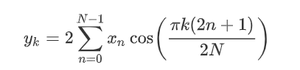Open in App
Not now

# Python – scipy.fft.idct() method

• Last Updated : 01 Oct, 2020

With the help of scipy.fft.idct() method, we can compute the inverse discrete cosine transform by selecting different types of sequences and return the transformed array by using this method.Syntax :

```scipy.fft.idct(x, type=2)
```

Return value: It will return the inverse transformed array.

Example #1: In this example, we can see that by using scipy.fft.idct() method, we are able to get the inverse discrete cosine transform by selecting different types of sequences by default it’s 2.

## Python3

 `# import scipy ` `from` `scipy ``import` `fft ` ` `  `# Using scipy.fft.idct() method ` `gfg ``=` `fft.idct([``0.1``, ``2.1``, ``0.3``, ``4.2``]) ` ` `  `print``(gfg)`

Output :

```[ 0.95238737 -0.80969772  0.7286317  -0.82132135]
```

Example #2:

## Python3

 `# import scipy ` `from` `scipy ``import` `fft ` ` `  `# Using scipy.fft.idct() method ` `gfg ``=` `fft.idct([``0.95238737``, ``-``0.80969772``, ``0.7286317``, ``-``0.82132135``], ``4``) ` ` `  `print``(gfg)`

Output :

```[0.12635568 0.17287895 0.19562573 0.51175513]
```
My Personal Notes arrow_drop_up
Related Articles